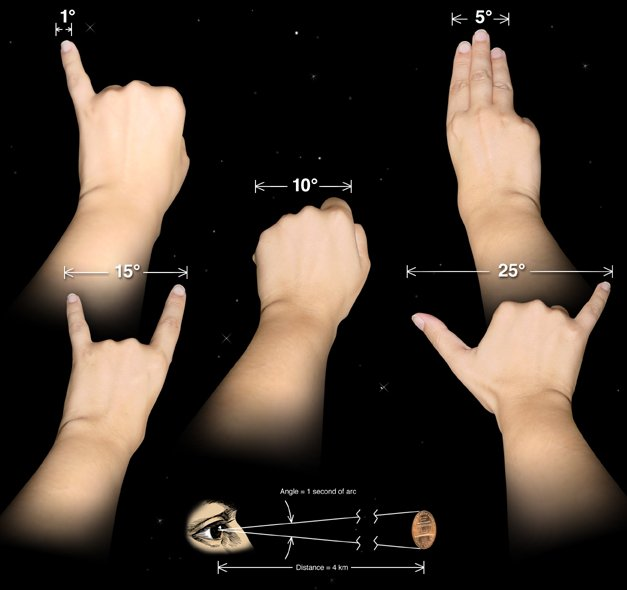A# angular diameterAngular diameter and linear diameter.How you can use your hand to make rough estimates of angular sizes. At arm's length, your little finger is about 1 degree across, your fist is about 10 degrees across, etc. Credit: NASA/ CXC/ M.Weiss.

Angular diameter is the angle that the actual diameter of an object makes in the sky; also known as angular size or apparent diameter. The Moon, with an actual diameter of 3,476 kilometers, has an angular diameter of 29' 21" to 33' 30", depending on its distance from Earth. If both angular diameter and distance are known, linear diameter can be easily calculated.

The Sun and the Moon have angular diameters of about half a degree, as would a 10-centimeter (4-inch) diameter orange at a distance of 11.6 meters (38 feet). People with keen eyesight can distinguish objects that are about an arc minute in diameter, equivalent to distinguishing between two objects the size of a penny at a distance of 70 meters (226 feet). Modern telescopes allow astronomers to routinely distinguish objects one arc second in diameter, and less. The Hubble Space Telescope, for example, can distinguish objects as small as 0.1 arc seconds. For comparison, 1 arc second is the apparent size of a penny seen at a distance of 4 kilometers (2.5 miles).

The angular diameter is proportional to the actual diameter divided by its distance. If any two of these quantities are known, the third can be determined.

For example if an object is observed to have an apparent diameter of 1 arc second and is known to be at a distance of 5,000 light years, it can be determined that the actual diameter is 0.02 light years.